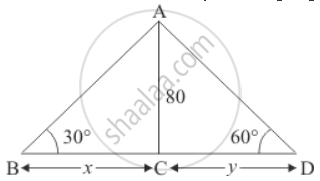Share

# Two Men on Either Side of the Cliff 80 M High Observe the Angles of an Elevation of the Top of the Cliff to Be 30° and 60° Respectively. Find the Distance Between the Two Men. - CBSE Class 10 - Mathematics

#### Question

Two men on either side of the cliff 80 m high observe the angles of an elevation of the top of the cliff to be 30° and 60° respectively. Find the distance between the two men.

#### Solution

Let AB and AD be the two men either side of cliff and height of cliff is 80 m.

And makes an angle of elevation, 30° and 60° respectively of the top of the cliff

We have given that AC = 80 m. Let BC = x and CD = y. And ∠ABC = 30°, ∠ADC = 60°

Here we have to find height of cliff.So we use trigonometric ratios

In a triangle ABC

=> tan B = (AC)/(BC)

=> tan 30^@ = 80/x

=> 1/sqrt3 = 80/x

=> x = 80sqrt3

=> tan D = (AC)/(CD)

=> tan  60^@ = 80/y

=> sqrt3 = 80/y

=> y = 80/sqrt3

=> x + y = 80sqrt3 + 80/sqrt3

=> x + y = 320/sqrt3

=> x + y = 184.8

Hence the height of cliff is 184.8 m

Is there an error in this question or solution?

#### Video TutorialsVIEW ALL 

Solution Two Men on Either Side of the Cliff 80 M High Observe the Angles of an Elevation of the Top of the Cliff to Be 30° and 60° Respectively. Find the Distance Between the Two Men. Concept: Heights and Distances.
S